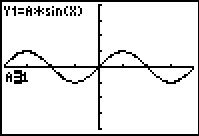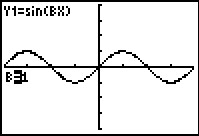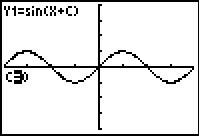# Activities

Vertical and Phase Shifts#### Activity Overview

Students explore vertical and phase shifts of sine and cosine functions.

#### Key Steps

•Students utilize the Transformation Graphing application to investigate the amplitude of trigonometric functions.

The program will graph the parent function y = sin(x) and define Y1 = a sin(x) into the screen. Students can enter different values for a and observe the effect on the function. They should find that the sine curve is vertically stretched by a factor of |a|.

•Next, students investigate the period of a trigonometric graph.

Here, Y1 = sin(bx), and students substitute given values for b, observing the effects on the function. They will find that the value of b affects the horizontal stretch of this function and thus changes the period.

•Students investigate a simple phase shift. The program will graph Y1 = sin(x + c) and students substitute given values of c to observe the shift.

Students then investigate a vertical shift. Investigating as before, students will find that the equation Y1 = sin(x) + d has a vertical shift equal to the parameter d.